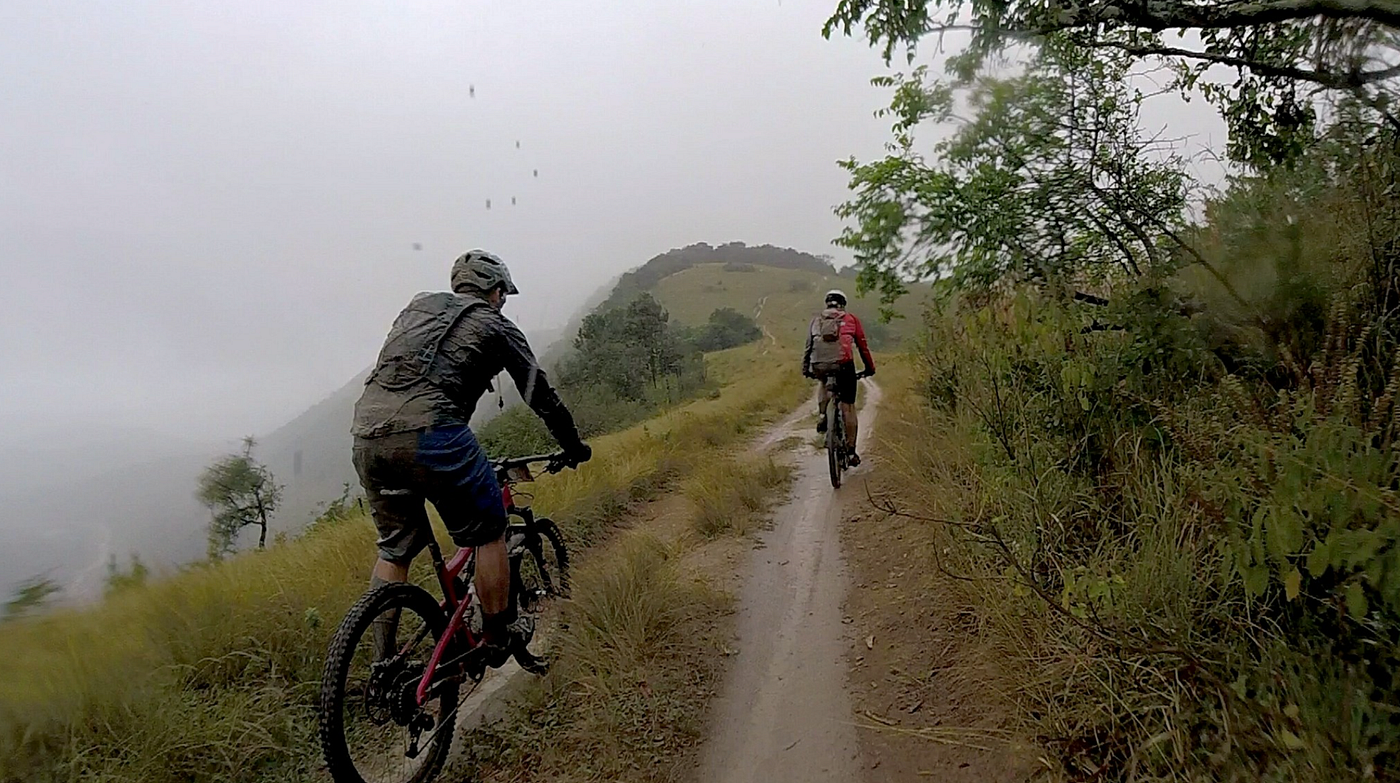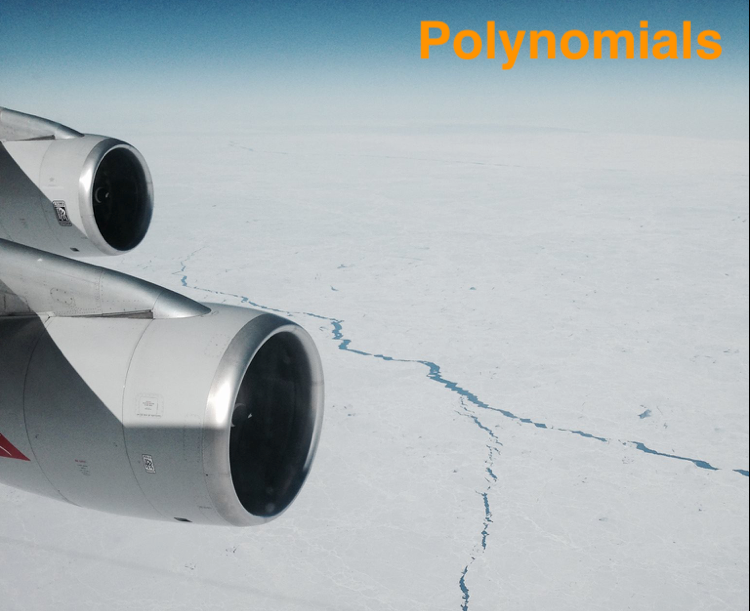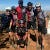# Introduction

This is a multi-class classification problem, meaning that there are more than two classes to be predicted, in fact there are 7 categories.

• If you would like to see how to code a neural network from scratch, check this article
• A very good article on multi class concepts which I reference below

`1. Import the classes and functions2. Train and save the model2.1 Load our data2.2 Prepare our features2.3 Split train and test data2.4 Hot Encoding Y2.5…`

# The Complete Guide to Neural Network multi-class Classification from scratch

## What on earth are neural networks? This article will give you a full and complete introduction to writing neural networks from scratch and using them for multinomial classification. Includes the python source code.

Neural networks reflect the behavior of the human brain. They allow programs to recognise patterns and solve common problems in machine learning. This is another option to either perform classification instead of logistics regression. At Rapidtrade, we use neural networks to classify data and run regression scenarios. The source code for this article is available on GitHub.

We will be working with a dataset from Kaggle and you can download it here. So to visualise the data we will be working with in this article, see below. We will use this to train the network to categorise our customers according…

# Linear regression: Comparing pythons sklearn with ‘writing it from scratch’

## Ever wanted to know what happens under the hood in multivariate linear regression/gradient descent in python’s sklearn? You will be surprised how easy it is. Lets write it from scratch and apply the same evaluation method to both to see how we do.Sani2C by author — could linear regression make me cycle faster?

# Introduction

So, understanding what happens in linear regression is so good from an understanding point of view. Here is a deep dive without using python libraries. Here is a link to the source code for this article in github.
If you do need an intro to gradient descent have a look at my 5 part YouTube series first.

In step 1, we will write gradient descent from scratch, while in step 2 we will use sklearn’s linear regression.

# Our dataset

Let’s download our dataset from kaggle. The Global Health Observatory (GHO) data repository under World Health Organization (WHO) keeps track of the health…

# The complete guide to Polynomials

## What on earth are polynomials? This article will give you a full and complete introduction to polynomials, including some source code.Source: Taken by Shaun Enslin, flying over Antartica 2014. I wonder what shape polynomial that would be?

# 1. Introduction

The problem with linear regression is that data does not often go in a straight line. If we look at below data set, a linear function makes perfect sense.

# Our dataset

Using PCA, we can reduce our features from (n) down to either 2 or 3 dimensions which can then be plotted. We will start by looking at our dataset as we downloaded from kaggle.

We can see 4 attributes, which is super for predictions, but does not allow us to plot a visualisation.

# Support Vector Machines — multinomial example

## SVM’s are great for classifying data you do see many binomial examples out there. Here is a good multinomial example in Matlab for you…

If you have arrived here and do not have a good understanding of SVM, then check this article first.

The Iris flower data set is a multivariate data set introduced by the British statistician and biologist Ronald Fisher in his 1936 paper The use of multiple measurements in taxonomic problems. It is sometimes called Anderson’s Iris data set because Edgar Anderson collected the data to quantify the morphologic variation of Iris flowers of three related species. The data set consists of 50 samples from each of three species of Iris (Iris Setosa, Iris virginica, and Iris versicolor). Four features were…

# The basics

The important job that SVM’s perform is to find a decision boundary to classify our data. This decision boundary is also called the hyperplane.

Lets start with an example to explain it. Visually, if you look at figure 1, you will see that it makes sense for purple line to be a better hyperplane than the black line. The black line will also do the job, but skates a little to close to one of the red points to make it a good decision line.

Visually, this is quite easy to spot.

# Implementing neural networks in matlab 105

Lets implement a neural network to classify customers according to their key features. Running neural networks in matlab is quite understandable once you understand the equations.

This is part 5 in my series on neural networks. You are welcome to start at part 1. Here are the previous articles explaining the cost function.

Source code used here can be downloaded from github. Spoiler alert, we will only get a 66% accuracy, but, thats the data we have ;-)

Just as a refresher, here is the dataset we downloaded from kaggle

# Neural networks cost and gradient calculation deep dive 104

After understanding forward and backward propagation, lets move onto calculating cost and gradient. This is vital component to neural networks.

This is part 2 in my series on neural networks. You are welcome to start at part 1 or skip to part 5 if you just want the code.

So, to perform gradient descent or cost optimisation, we need to write a cost function which performs:

In this article we will deal with (3) and (4). You can click on the links above for a deep dive on forward/back prop.

So, just…

# Neural networks backward propagation deep dive 103

Backward propagation is a tricky subject to explain. Lets give it a go here showing the code and output data as we go.

This is part 3 in my series on neural networks. You are welcome to start at part 1 or skip to part 5 if you just want the code.

So, to perform gradient descent or cost optimisation, we need to write a cost function which performs: